# How do we describe motion around us?From rotating galaxies to jiggling atoms, the primary and most beautiful of nature's qualities is motion, which agitates her at all times. Without motion, it is very hard to imagine of life. Without motion, neither you nor I would exist!

We can understand the type of motion from our knowledge of position, distance, displacement, speed, velocity and acceleration. This little branch of mechanics in which we study how things move without knowing the cause of their motion is called kinematics.

Why study motion?

When a cricket match is telecast live on tv, you are told about many things such as the speed at which the ball was bowled, hitting speed of the batsman, six distance, reaction time of the fielder, trajectories followed by the ball and so on.paths followed by the ball

Similarly, in other games, such as badminton, football or tennis, kinematics is applicable in real time. Therefore, we can start to appreciate many aspects of the game by knowing the motions of different particles involved in the game.

Consider another example: when a car is moving and suddenly brakes are applied, you may have noticed that the car stops completely only after covering certain distance. Therefore, automobile engineers want to prevent accidents by reducing this distance.

What is moving?

You could say that the road does not move or the table in your room is at rest. But even these objects are moving because the earth is moving! The earth rotates about its axis as well as revolves around the sun.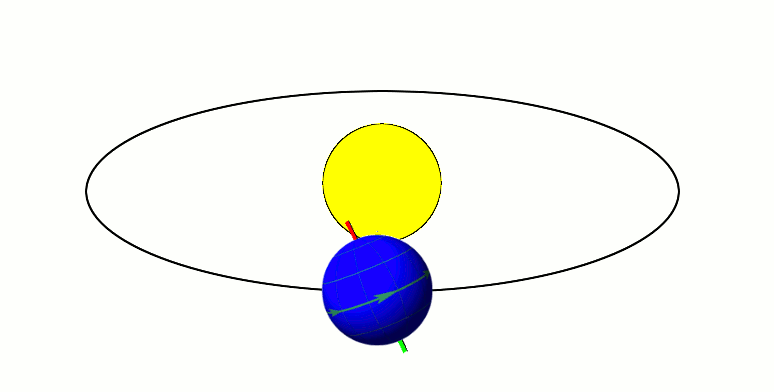Let's understand this with example: suppose you are sitting inside a moving bus. Then, from your perspective, the passengers appear motionless in the same way as the table in the room. But for someone waiting at the bus stop, the bus as well as the commuters inside it, are in motion.

In similar way, everything in our immediate surroundings may appear to be at rest, but from a larger perspective, such as from outer space, let's say, the earth is moving and therefore, everything on it is moving as well!

Definition

An object is in motion with respect to an observer if it occupies different positions at different instants of time as measured by that observer.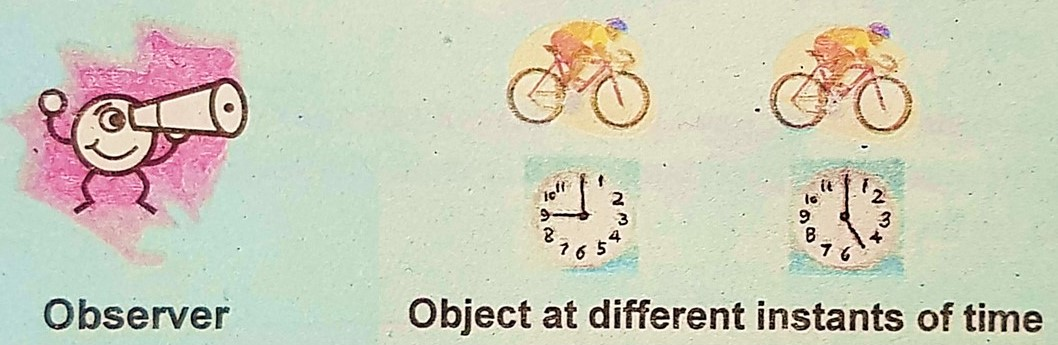Thus, whenever you deal with motion, you should keep three questions in mind: where? when? and with respect to what, or with respect to whom? Once you have the three answers, you can work out a large number of problems in kinematics.

Solved  question
In the animation shown below, which objects are at rest and which ones are in motion, with respect to whom or what?If we assume ground our stationary reference point, then, with respect to the ground, the horse is moving. As a result, man is also moving with respect to the ground. From the perspective of the horse, man is at rest. Therefore, with respect to man, the horse is too at rest.

Position time graph

Consider a car moving on straight road. From our definition, the location of the car is function of time. Therefore, let us draw horizontal and vertical axes to represent time and position respectively, as shown below.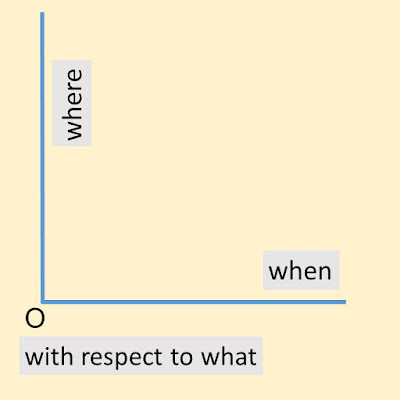In the picture, the vertical line is to tell us where the car is situated on the road, the horizontal line is to tell us when, and O is the reference point or origin, meaning, that we measure the journey of the car with respect to it.

Solved  question
In the position-time graph shown below, what is the position of the moving particle at t=3 seconds and t=5 seconds?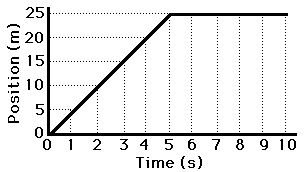At t=3 seconds, the particle is situated at 15 meters from the origin. At t=5 seconds, the particle is at 25 m. The particle comes to stop and its position does not change after t=5 seconds.

Distance

Suppose the car goes from point A to point B on the road. Then, it turns around, goes from point B to point C and stops there. Let us draw the movement of the car on the position-time diagram, as shown below.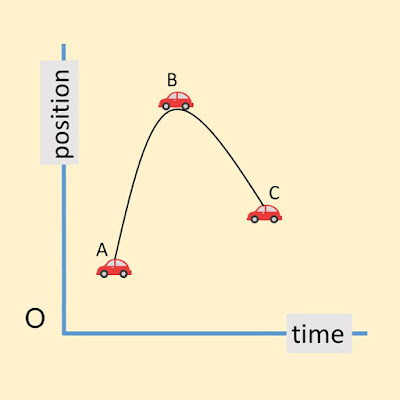Notice: the car is not moving along the curve but it is actually moving on the vertical road. Since the position of the car is function of time, the curve could be traced by "joining the dots" of the journey.

What is the total distance travelled by the car? It is pretty clear when we look at the vertical road. The car went from point A to point B and from point B to point C. Therefore, the total distance covered by the car is AB+BC.Remember: the total distance covered by moving object is obtained by ordinary addition. This means that the car may run back and forth on the straight road but the distance will never decrease, that is, always positive.

Solved  question
The international space station orbits the earth 16 times in one day at a distance of 400 km from the surface. Calculate the total distance covered by the ISS in one day if radius of the earth is 6,400 km.First, we must calculate the radius of the orbit, that is, the distance from the center of the earth to the station. This is simply, 6400+400=6800 km, as shown in the diagram below: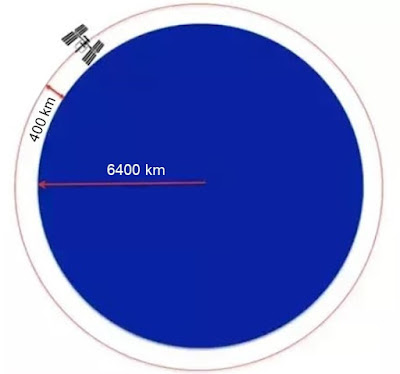The circumference of a circle is 2πr, which is, (2×3.14×6800) = 42,704 km. This is the distance covered in one orbit. Thus, in one day, the space station goes total distance: 16×42,704 = 683,264 km.

Position vector

The location of the moving car with respect to the origin can be represented by an arrow pointing from the origin to the position of the car. One end of the arrow is fixed while the other end is attached to the moving car.The arrow is called vector, having two properties: length and direction. You can tell the type of motion from observing the behaviour of the position vector:

• If the length and direction of the position vector do not change in time with respect to the origin, then the object is not moving.
• If the length of the position vector changes but not its direction, then the object is moving in straight line, as in our case.
• If the direction of the position vector changes but not its length, then the object is moving in two-dimensional circular motion.
• When both the length as well as the direction of the position vector change, the object is moving in complicated multi-dimensional motion.

Displacement

Let's draw the position vectors OC and OA to indicate the final and initial positions of the car with respect to the origin, respectively. Then, the displacement of the car would be the vector difference OCOA, that is, final minus initial position.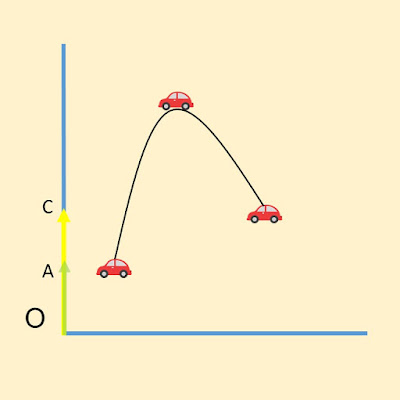The difference of two vectors is also vector. Therefore, displacement is a vector quantity, pointing from the initial position of the object to its final position. In our case, from A to C, as shown below.

The length of the arrow AC is the amount by which the car has been displaced or moved. Therefore, displacement is the change in position of the moving object. The tip of the arrow AC is the direction of the displacement.Alternatively: what if the car had returned back to the initial position A instead of stopping at point C? Then, the displacement of the car for the total journey would be OAOA, that is, zero!

Consider yet another situation: the car does not stop at point C, but instead, goes further up to point D, as shown in the position-time diagram below. What is then the displacement of the car?By using the same concept again, final minus initial position, the displacement of the car would be ODOA, where OD and OA are final and initial position vectors of the car on the vertical road, respectively:The length of vector OD is less than that of vector OA. Therefore, the displacement, ODOA will be negative. It's like subtracting 4 from 2. Physically, it means that the car ran backwards, from A to D, as shown: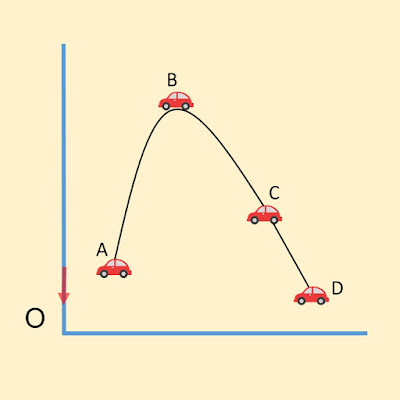Thus, while the total distance covered by moving object is always positive, the displacement, on the other hand, could be positive, negative or zero. Both distance and displacement are lengths, measured in the same units, and yet different in a very special way.

Solved  question
Look at the position-time graph of a bike moving on straight road. Find the displacement of the bike between t=5 seconds and t=15 seconds.At t=15 seconds, final position is x=0 m from the origin. At t=5 seconds, initial position is x=20 m from the origin. Displacement is change in position. Therefore, final minus initial position= –20 m.

Speed

Usain Bolt holds the world record for running 100 meters in 9.58 seconds. The speed of a moving object is the measure of how fast it goes. In this example, Bolt ran at the speed of about 10.44 meters per second, which is pretty fast for any human.However, Bolt didn't run at same rate the whole time. In the beginning, he was picking up speed, that is, going at a rate far less than the average 10.44 m/s. He ran at his fastest in the middle of the race, and slowed towards the end.

Thus, we have two types of speed: average and instantaneous. The average speed is the rate at which the overall journey took place. The instantaneous speed, on the other hand, is real-time measurement, as shown in the speed-time graph below.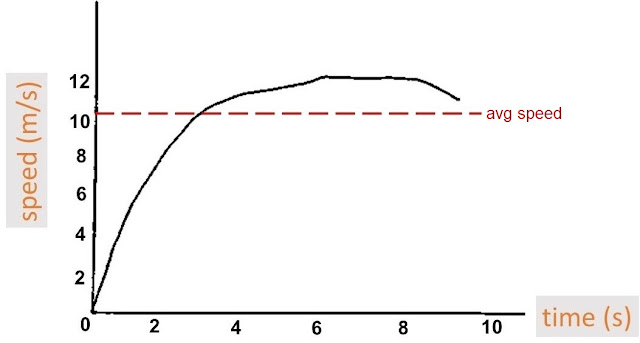It is obvious from the graph that average speed does not provide much info about the actual motion or variation that occurred throughout the journey. On the contrary, instantaneous speed is the true measure of the motion of the body.

Solved  question
Light from the sun takes about 8 minutes and 20 seconds to reach the earth surface. The distance between sun and earth is 150 million km. Calculate the speed of light in m/s.Speed is distance divided by time. The time taken is 500 seconds. The distance between the two bodies is 150,000,000,000 meters. Therefore, the speed of light is 150,000,000,000/500= 3×10^8 m/s.

Velocity

In day-to-day life, speed and velocity are often used interchangeably. However, in physics, they are separate physical quantities. Speed is the measurement of rate of motion whereas velocity is the measurement of rate as well as direction of motion.

Consider the image below. Suppose, the rate at which each vehicle is going, that is, their speed, is the same. How can then we differentiate the ones moving on the left from the ones moving on the right? We use the concept of direction for this very purpose.The vehicles on the right are moving, let's say, northwards. Then, the others, on the left, are heading south. Thus, even when their speeds are the same, their velocities are different. You can say, velocity is speed with direction.

In other words, velocity of a moving object can change in three ways: 1) when speed or magnitude changes, but direction does not, 2) when speed remains constant, however, direction changes and 3) when both speed and direction change.

Average & instantaneous velocity

Just like instantaneous speed, instantaneous velocity is also the real-time measurement of how fast the object is going. It's measured in the same units, m/s or kph. However, now, we also have the sense of direction, that is, where the object is headed.

For example, anemometer is a common weather station instrument, which is used to measure instantaneous velocity (speed and direction) of the wind. Anemometers are important tools for meteorologists, who study the weather patterns.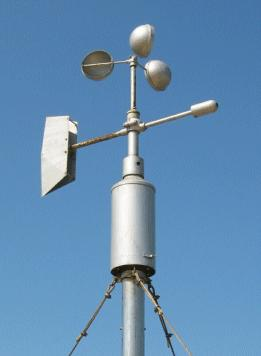Average velocity is, once again, the overall measurement of the journey. It is different from average speed though, defined as: total displacement divided by total time. Since the total displacement could be positive, negative or zero, average velocity can also be the same.

It is important to note that physicists hardly use the concept of average velocity because instantaneous velocity does a pretty good job on its own. And when it's required to measure the overall rate of motion, average speed outranks average velocity.

Still, to determine the overall direction of motion, average velocity is pretty handy. More specifically, the sign of average velocity (+ or –) is to tell us the direction in which the object was headed. The following three situations exist:

1. When average velocity is zero: this is when the displacement of the object is zero, that is, it has returned back to its original location after finishing the journey2. When average velocity is positive: this is when the displacement of the object is positive, that is, it has displaced (or moved) away from the original location in the positive direction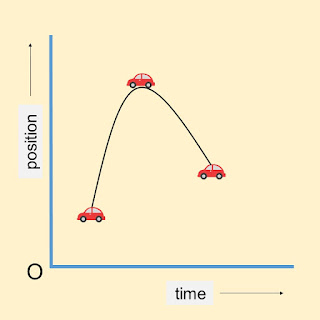3. When average velocity is negative: this is when the displacement of the object is negative, that is, it has gone past the original location, in the opposite direction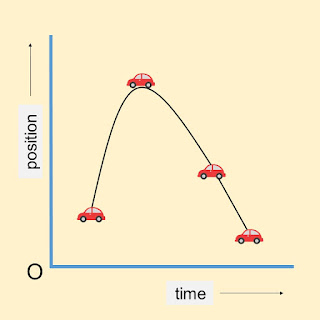Like average velocity, instantaneous velocity could also be positive, negative or zero. However, there is a key difference: While the average is helpful to determine the overall direction, instantaneous velocity is the true measure of direction at any instant of time.

Velocity time graph

Suppose, you throw a tennis ball upwards, and it goes in straight line. Let's say the up direction, is positive. As it goes upwards, the velocity of the ball continues to decrease, why, because it slows down due to the effects of gravity.

When it reaches the top, the velocity of the ball would be zero, at least for a brief moment. Then, the ball starts falling, again due to gravity, in the negative direction. As it falls, the velocity of the ball continues to increase. The velocity-time graph is shown below: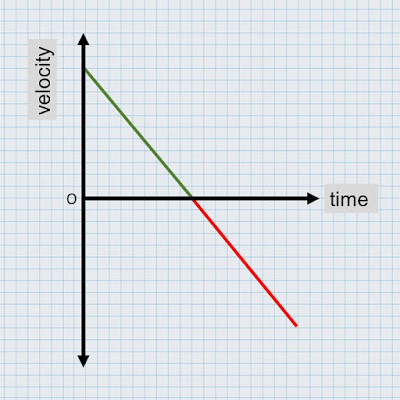Look at the graph: the part in the green is when the ball is going upwards. The velocity of the ball is losing the magnitude, then it hits the zero, that is, when the ball is at the top. Then, the part in the red is when the ball descends. The magnitude of the velocity duplicates itself in the negative direction.

What if we chose the upward direction, negative, and the direction downward, positive? It is important to understand that the choice is completely ours. The numbers will not change, but, the signs definitely will. The graph will too, as shown below.There is no change in the meanings of green and red parts. The green is still when the ball ascends and the red is when it descends. It is important that once you have made the choice of directions, you stick to it for the rest of the question, experiment, or what have you.

Acceleration

Many people like to discuss acceleration of different vehicles just to see which one is better at picking up speed. Let's say, a bike can go from 0 kph to 100 kph in 10 seconds. There's another bike which can achieve the same result in only 4 seconds. Pretty cool, right?

In physics, acceleration is defined as the rate of rate of motion, that is, how fast (or slow) the object can switch between two velocities. The formula is simply, change in velocity divided by total time taken. It is measured in m/s².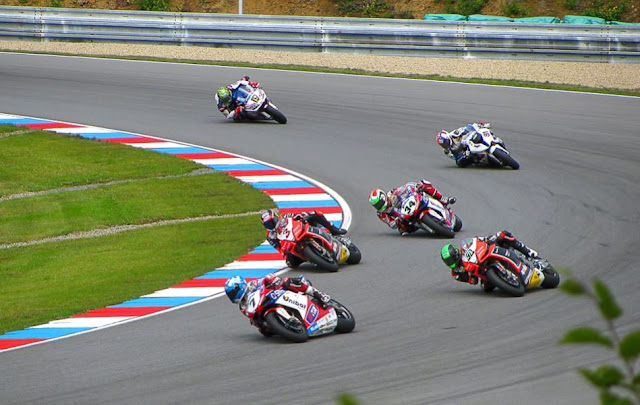When does a moving body undergo acceleration? Since acceleration is dependent on change in velocity, there can be three cases: 1) when the speed changes (increase or decrease) but direction remains constant, 2) when the speed is constant but the direction is not, as in, uniform circular motion and 3) when both speed and direction change

Solved  question
Given below is velocity-time graph for 4 objects moving in straight line. Which object is accelerating and which is not? Lastly, which object is decelerating, that is, slowing down? Let's say the initial velocity of object B is 18 m/s and after 10 seconds, its velocity increased to 38 m/s, what is the acceleration of object B?Velocities of objects B and D are increasing with time, therefore, they are both accelerating. On the other hand, the velocity of object C is constant in time, its acceleration is zero. Lastly, object A is decelerating, as it is undergoing reduction in velocity.

To find the acceleration of object B, use the formula change in velocity divided by time taken. Given, the change in velocity is 38–18 = 20 m/s. The time taken to achieve this change is 10 s. Therefore, acceleration is 20÷10 = 2 m/s².

Summing up

You now have the understanding how things move. Also, you have been introduced to vector quantities in physics. The key terms: position, displacement, speed, velocity and acceleration, hopefully make sense. This is it, folks! In the next post of kinematics, we will see how displacement, velocity and acceleration are intimately related to one another.